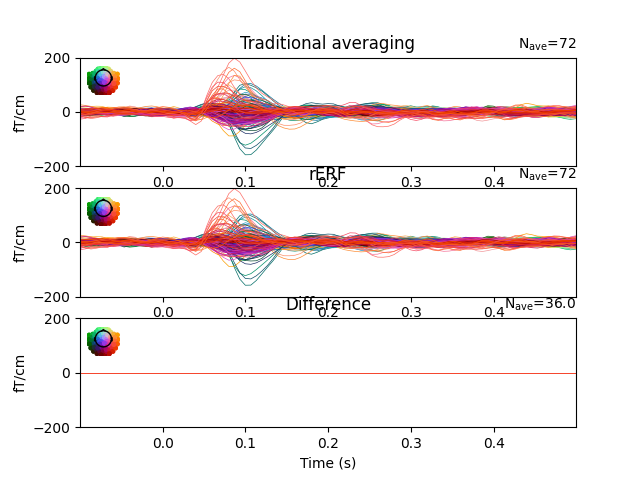# Regression on continuous data (rER[P/F])#

This demonstrates how rER[P/F]s - regressing the continuous data - is a generalisation of traditional averaging. If all preprocessing steps are the same, no overlap between epochs exists, and if all predictors are binary, regression is virtually identical to traditional averaging. If overlap exists and/or predictors are continuous, traditional averaging is inapplicable, but regression can estimate effects, including those of continuous predictors.

rERPs are described in Smith and Kutas.

```# Authors: Jona Sassenhagen <jona.sassenhagen@gmail.de>
#
```
```import matplotlib.pyplot as plt

import mne
from mne.datasets import sample
from mne.stats.regression import linear_regression_raw

data_path = sample.data_path()
meg_path = data_path / "MEG" / "sample"
raw_fname = meg_path / "sample_audvis_filt-0-40_raw.fif"
raw.filter(1, None, fir_design="firwin")  # high-pass

# Set up events
events = mne.find_events(raw)
event_id = {"Aud/L": 1, "Aud/R": 2}
tmin, tmax = -0.1, 0.5

# regular epoching
picks = mne.pick_types(raw.info, meg=True)
epochs = mne.Epochs(
raw,
events,
event_id,
tmin,
tmax,
reject=None,
baseline=None,
verbose=False,
)

# rERF
evokeds = linear_regression_raw(
raw, events=events, event_id=event_id, reject=None, tmin=tmin, tmax=tmax
)
# linear_regression_raw returns a dict of evokeds
# select conditions similarly to mne.Epochs objects

# plot both results, and their difference
cond = "Aud/L"
fig, (ax1, ax2, ax3) = plt.subplots(3, 1)
params = dict(
)
epochs[cond].average().plot(axes=ax1, **params)
evokeds[cond].plot(axes=ax2, **params)
contrast = mne.combine_evoked([evokeds[cond], epochs[cond].average()], weights=[1, -1])
contrast.plot(axes=ax3, **params)
ax2.set_title("rERF")
ax3.set_title("Difference")
plt.show()
``````Opening raw data file /home/circleci/mne_data/MNE-sample-data/MEG/sample/sample_audvis_filt-0-40_raw.fif...
Read a total of 4 projection items:
PCA-v1 (1 x 102)  idle
PCA-v2 (1 x 102)  idle
PCA-v3 (1 x 102)  idle
Average EEG reference (1 x 60)  idle
Range : 6450 ... 48149 =     42.956 ...   320.665 secs
Removing projector <Projection | PCA-v1, active : False, n_channels : 102>
Removing projector <Projection | PCA-v2, active : False, n_channels : 102>
Removing projector <Projection | PCA-v3, active : False, n_channels : 102>
Removing projector <Projection | Average EEG reference, active : False, n_channels : 60>
Reading 0 ... 41699  =      0.000 ...   277.709 secs...
Filtering raw data in 1 contiguous segment
Setting up high-pass filter at 1 Hz

FIR filter parameters
---------------------
Designing a one-pass, zero-phase, non-causal highpass filter:
- Windowed time-domain design (firwin) method
- Hamming window with 0.0194 passband ripple and 53 dB stopband attenuation
- Lower passband edge: 1.00
- Lower transition bandwidth: 1.00 Hz (-6 dB cutoff frequency: 0.50 Hz)
- Filter length: 497 samples (3.310 s)

[Parallel(n_jobs=1)]: Done  17 tasks      | elapsed:    0.0s
[Parallel(n_jobs=1)]: Done  71 tasks      | elapsed:    0.2s
[Parallel(n_jobs=1)]: Done 161 tasks      | elapsed:    0.4s
319 events found on stim channel STI 014
Event IDs: [ 1  2  3  4  5 32]
```

Total running time of the script: (0 minutes 5.718 seconds)

Estimated memory usage: 194 MB

Gallery generated by Sphinx-Gallery